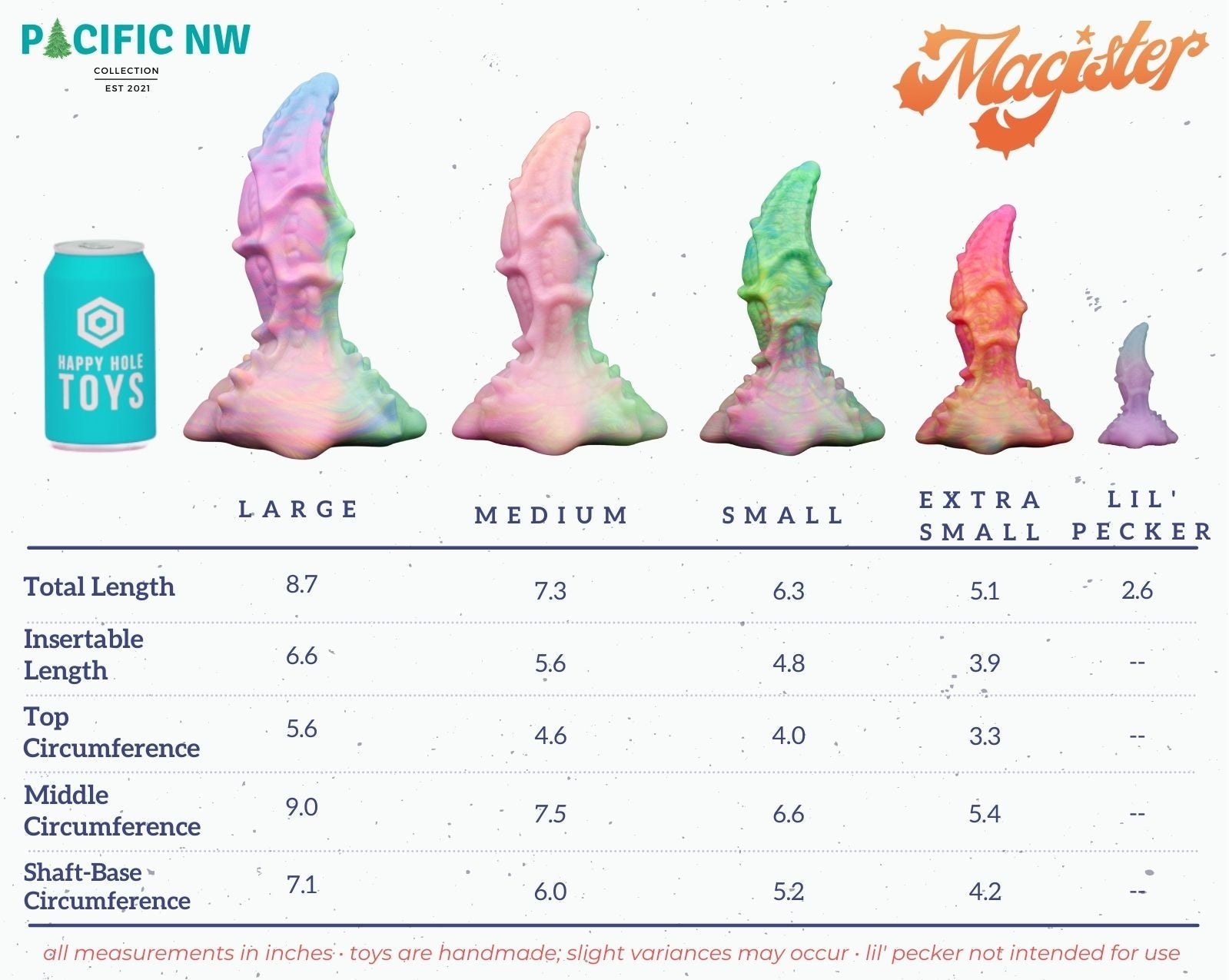# Magister Size Chart

Text-reader friendly Magister size information can be found after this image:OCR-friendly Magister size information:

• Magister XSmall: Total Length is 5.1 inches and the Insertable Length is 3.9 inches. The Top Circumference is 3.3 inches, the Middle Circumference is 5.4 inches, and the Shaft-Base Circumference is 4.2 inches
• Magister Small: Total Length is 6.3 inches and the Insertable Length is 4.8 inches. The Top Circumference is 4.0 inches, the Middle Circumference is 6.6 inches, and the Shaft-Base Circumference is 5.2 inches.
• Magister Medium: Total Length is 7.3 inches and the Insertable Length is 5.6 inches. The Top Circumference is 4.6 inches, the Middle Circumference is 7.5 inches, and the Shaft-Base Circumference is 6.0 inches.
• Magister Large: Total Length is 8.7 inches and the Insertable Length is 6.6 inches. The Top Circumference is 5.6 inches, the Middle Circumference is 9.0 inches, and the Shaft-Base Circumference is 7.1 inches.